# MCQ in Electrical Circuit Part 17 | ECE Board Exam

(Last Updated On: November 24, 2020)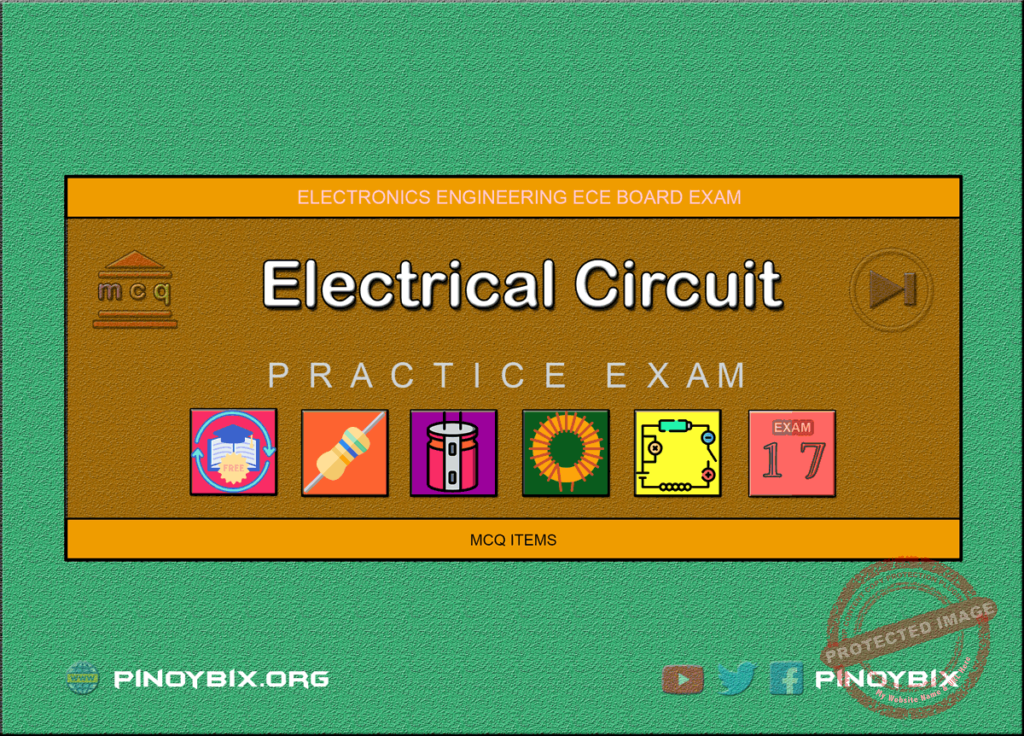This is the Multiples Choice Questions Part 17 of the Series in Electrical Circuit as one of the Electronics Engineering topic. In Preparation for the ECE Board Exam make sure to expose yourself and familiarize in each and every questions compiled here taken from various sources including but not limited to past Board Exam Questions in Electronics Engineering field, Electronics Books, Journals and other Electronics References.

#### MCQ Topic Outline included in ECE Board Exam Syllabi

• MCQ in AC-DC circuits
• MCQ in Resistors
• MCQ in Inductors
• MCQ in Capacitors

#### Continue Practice Exam Test Questions Part 17 of the Series

Choose the letter of the best answer in each questions.

801. Four heaters having the same voltage rating will produce maximum heat if connected in

A. Series

B. Parallel

C. Series-Parallel

D. Parallel-Series

View Answer:

Answer: Option B

Solution:

802. 1000 kW is equal to how many is ergs/sec.

A. 2 x 10^13

B. 1 x 10^16

C. 1 x 10^13

D. 2 x 10^10

View Answer:

Answer: Option C

Solution:

803. When current flows through heater coil, it glows but supply wiring does not glow because

A. supply wiring is covered with insulation wiring

B. current through supply line flows at slower speed

C. supply wires are made of superior material

D. resistance of heater coil is more than that of supply wire

View Answer:

Answer: Option D

Solution:

804. REE Board Exam April 1997

A circuit consisting of three resistors rated: 10 ohms, 15 ohms and 20 ohms are connected in delta. What would be the resistance of the equivalent wye connected load?

A. 0.30, 0.23 & 0.15 ohm

B. 3.0, 4.0 & 5.0 ohms

C. 3.33, 4.44 & 6.66 ohms

D. 5.77, 8.66 & 11.55 ohms

View Answer:

Answer: Option C

Solution:

805. EE Board Exam October 1994

The equivalent wye element of a 3 equal resistors each equal to R and connected in delta is

A. R

B. 3R/2

C. R/3

D. 3R

View Answer:

Answer: Option C

Solution:

806. EE Board Exam April 1988

A Barangay power station supplies 60 kW to a load over 2,500 ft, 100 mm^2, two-conductor copper feeder, the resistance of which is 0.078 ohm per 1000 ft. The bus bar voltage is maintained constant at 600 V. Determine the load current.

A. 105 A

B. 108 A

C. 110 A

D. 102 A

View Answer:

Answer: Option B

Solution:

807. EE Board Exam October 1986

An LRT car, 5 km distance from the Tayuman station, takes 100 A over a 100 mm hard drawn copper trolley wire having a resistance of 0.270 ohm per km. The rail and ground return has a resistance of 0.06 ohm per km. If the station voltage is 750 V, what is the voltage of the car?

A. 585 V

B. 590 V

C. 595 V

D. 580 V

View Answer:

Answer: Option A

Solution:

808. EE Board Exam April 1989

The LRT trolley system 10 miles long is fed by two substations that generate 600 volts and 560 volts, respectively. The resistance of the trolley wire and rail return is 0.3 ohm per mile. If the car is located 4 miles from the 600 volt station draws 200 A from the line. How much is the current supplied by each station?

A. 133.33 A, 66.67 A

B. 123.67 A, 76.33 A

C. 117.44 A, 82.56 A

D. 125.54 A, 63.05 A

View Answer:

Answer: Option A

Solution:

809. EE Board Exam April 1992

In Manila, the LRT runs between Gil Puyat Station and Tayuman Station, which is 4 km apart and maintains voltages of 420 volts and 410 volts respectively. The resistance of go and return is 0.05 ohm per km. The train draws a constant current of 300 A while in motion. What are the currents supplied by the two stations if the train is at the distance of minimum potential?

A. 175 A, 125 A

B. 183 A, 117 A

C. 164 A, 136 A

D. 172 A, 123 A

View Answer:

Answer: Option A

Solution:

810. EE Board Exam October 1986

An LRT car, 5 km distance from the Tayuman station, takes 100 A over a 100 mm hard drawn copper trolley wire having a resistance of 0.270 ohm per km. The rail and ground return has a resistance of 0.06 ohm per km. If the station voltage is 750 V, what is the efficiency of transmission?

A. 78%

B. 81%

C. 74%

D. 79%

View Answer:

Answer: Option A

Solution:

811. EE Board Exam April 1988

A barangay power station supplies 60 kW to a load over 2,500 ft of 0002-conductor copper feeder the resistance of which is 0.078 ohm per 1,000 ft. The bus bar voltage is maintained constant at 600 volts. Determine the maximum power which can be transmitted.

A. 220.35 kW

B. 230.77 kW

C. 242.73 kW

D. 223.94 kW

View Answer:

Answer: Option B

Solution:

812. EE Board Exam April 1991

Twelve similar wires each of resistance 2 ohms are connected so as to form a cube. Find the resistance between the two diagonally opposite corners.

A. 1.45 ohms

B. 1.66 ohms

C. 2.01 ohms

D. 1.28 ohms

View Answer:

Answer: Option B

Solution:

813. EE Board Exam April 1991

Twelve similar wires each of resistance 2 ohms are connected so as to form a cube. Find the resistance between the two corners of the same edge.

A. 1.133 ohms

B. 1.102 ohms

C. 1.125 ohms

D. 1.167 ohms

View Answer:

Answer: Option D

Solution:

814. EE Board Exam October 1991

Twelve identical wires each of resistance 6 ohms are arranged to form the edge of a cube. A current of 40 mA is led into the cube at one corner and out at the other diagonally opposite corners. Calculate the potential difference developed between these corners.

A. 0.20 V

B. 0.28 V

C. 0.22 V

D. 0.24 V

View Answer:

Answer: Option A

Solution:

815. EE Board Exam August 1976

Find the value of the voltage V.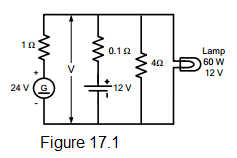electrical circuit figure 17.1

A. 12.34 V

B. 11.24 V

C. 12.19 V

D. 11.66 V

View Answer:

Answer: Option A

Solution:

816. EE Board Exam April 1982

Referring to the circuit diagram below, if the charger voltage is 130 volts and the battery voltage is 120 volts, solve for the current Ib.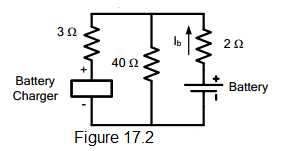electrical circuit figure 17.2

A. -0.215 A

B. 0.215 A

C. -0.306 A

D. 0.306 A

View Answer:

Answer: Option A

Solution:

817. EE Board Exam August 1977

In the figure below R1 = 1 ohm, R2 = 1 ohm, R3 = 3 ohms, I2 = 2 A and VB = 120 V. Find Eg.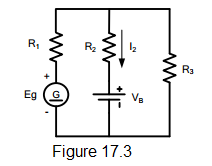electrical circuit figure 17.3

A. 182.41 V

B. 153.32 V

C. 164.67 V

D. 157.22 V

View Answer:

Answer: Option C

Solution:

818. EE Board Exam October 1980, April 1984

In the dc circuit as shown, the high resistance voltmeter gives a reading of 0.435 volt. What is the value of the resistance R?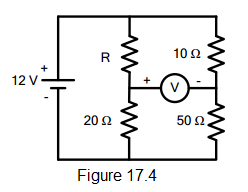electrical circuit figure 17.4

A. 4 ohms

B. 5 ohms

C. 3 ohms

D. 2 ohms

View Answer:

Answer: Option C

Solution:

819. EE Board Exam April 1980

Determine I in the figure.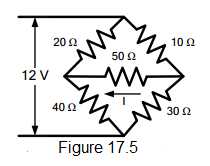electrical circuit figure 17.5

A. 0.028 A

B. 0.010 A

C. 0.025 A

D. 0.014 A

View Answer:

Answer: Option D

Solution:

820. ECE Board Exam April 1999

In Kirchhoff’s current law, which terminal of a resistance element is assumed to be at a higher potential (more positive) than the other?

A. The terminal where the current exits the resistance elements

B. The terminal where the current enters the resistance elements

C. Either A or B can be arbitrarily selected

D. The terminal closest to the node being analyzed

View Answer:

Answer: Option B

Solution:

821. ECE Board Exam April 2000

According to Kichhoff’s current law, what is the algebraic sum of all currents entering and exiting a node.

A. zero

B. a negative value

C. the algebraic sum of all currents

D. a positive value

View Answer:

Answer: Option A

Solution:

822. ECE Board Exam November 1997

Find the Thevenin’s impedance equivalent across R2 of a linear close circuit having 10 volts supply in series with the resistors (R1 = 100 ohms and R2 = 200 ohms).

A. 6.66 ohms

B. 6.666 kohms

C. 66.6 ohms

D. 666 ohms

View Answer:

Answer: Option C

Solution:

823. ECE Board Exam April 1999

What is a node?

A. A terminal point for a loop current

B. A connection point between two or more conductors

C. A formula

D. A mathematical fiction

View Answer:

Answer: Option B

Solution:

824. ECE Board Exam November 1998

If a resistance element is part of two loops, how many voltage drops must be calculated for that component?

A. Two

B. Three

C. One

D. None

View Answer:

Answer: Option A

Solution:

825. ECE Board Exam April 1998

How many nodes are needed to completely analyze a circuit according to Kirchhoff’s current law?

A. One

B. Two

C. One less than the total number of nodes in the circuit

D. All nodes in the circuit

View Answer:

Answer: Option D

Solution:

826. ECE Board Exam November 1996

Find the Thevenin’s impedance equivalent across R2 of a linear close circuit having 10 volt supply in series with two resistors (R1=50 ohms and R2 = 200 ohms).

A. 400 ohms

B. 40 ohms

C. 4 ohms

D. 4 kohms

View Answer:

Answer: Option B

Solution:

827. ECE Board Exam November 1995

In order to match the load to the generator means making load resistance __________.

A. lower than generator’s internal resistance

B. increased to more generator’s internal resistance

C. decreased

D. equal to generator’s internal resistance

View Answer:

Answer: Option D

Solution:

828. A circuit contains a 5 A current source in parallel with an 8 ohm resistor. What is the Thevenin’s voltage and Thevenin’s resistance of the circuit?

A. 40 V, 8 ohms

B. 5/8 V, 40 ohms

C. 5 V, 8 ohms

D. 5/8 V, 8 ohms

View Answer:

Answer: Option A

Solution:

829. In the Norton’s equivalent circuit, the source is a

A. constant voltage source

B. constant current source

C. constant voltage, constant current

D. none of these

View Answer:

Answer: Option B

Solution:

830. The superposition theorem requires as many circuits to be solved as there are

A. meshes

B. source

C. nodes

D. all of these

View Answer:

Answer: Option B

Solution:

831. Three resistors of 6-ohm resistance are connected in delta. Inside the delta another 6-ohm resistors are connected in wye. Find its resistance between any two corners.

A. 2 ohms

B. 3 ohms

C. 4 ohms

D. 1 ohm

View Answer:

Answer: Option C

Solution:

832. A 2-wire dc distribution line has sending end voltage of 240 V and total line resistance of 0.4 ohm. The maximum kW that can be transmitted by the line is ________.

A. 108

B. 72

C. 36

D. 144

View Answer:

Answer: Option C

Solution:

833. An active element in a circuit is one which _________.

A. receives energy

B. supplies energy

C. both receives and supplies energy

D. none of these

View Answer:

Answer: Option B

Solution:

834. An passive element in a circuit is one which __________.

A. supplies energy

B. receives energy

C. both receives and supplies energy

D. none of these

View Answer:

Answer: Option B

Solution:

835. An electric circuit contains __________.

A. active elements only

B. passive element only

C. both active and passive elements

D. none of these

View Answer:

Answer: Option C

Solution:

836. A linear circuit is one whose parameters (e.g. resistances etc.) ___________.

A. change with change in current

B. change with change in voltage

C. do not change with change in voltage and current

D. none of these

View Answer:

Answer: Option C

Solution:

837. In the circuit shown in Fig. 17.6, the number of nodes is __________.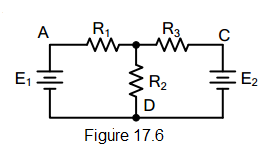electrical circuit figure 17.6

A. one

B. two

C. three

D. four

View Answer:

Answer: Option B

Solution:

838. In the circuit shown in Fig. 17.6, there are _________ junctions.electrical circuit figure 17.6

A. three

B. four

C. two

D. none of these

View Answer:

Answer: Option B

Solution:

839. The circuit shown in Fig. 17.6 has __________ branches.electrical circuit figure 17.6

A. two

B. four

C. three

D. none of these

View Answer:

Answer: Option C

Solution:

840. The circuit shown in Fig. 17.6 has __________ loops.electrical circuit figure 17.6

A. two

B. four

C. three

D. none of the above

View Answer:

Answer: Option C

Solution:

841. In the circuit shown in Fig. 17.6, there are ________ meshes.electrical circuit figure 17.6

A. two

B. three

C. four

D. none of these

View Answer:

Answer: Option A

Solution:

842. To solve the circuit shown in Fig. 17.7 by Kirchhoff’s laws, we require ________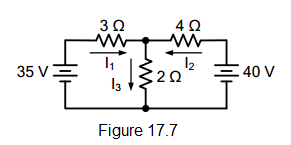electrical circuit figure 17.7

A. one equation

B. two equations

C. three equations

D. four equations

View Answer:

Answer: Option C

Solution:

843. To solve the circuit shown in Fig. 17.7 by nodal analysis, we require ________electrical circuit figure 17.7

A. one equation

B. two equation

C. three equations

D. none of these

View Answer:

Answer: Option A

Solution:

844. To solve the circuit shown in Fig. 17.7 by superposition theorem, we require ________electrical circuit figure 17.7

A. one circuit

B. two circuits

C. three circuits

D. none of these

View Answer:

Answer: Option B

Solution:

845. To solve the circuit shown in Fig. 17.7 by Maxwell’s mesh current equation, we requireelectrical circuit figure 17.7

A. one equation

B. three equations

C. two equations

D. none of these

View Answer:

Answer: Option C

Solution:

846. In the circuit shown in Fig. 17.8, the voltage at node B with D is calculated to be 15 V. The current in the 3 Ω resistor will be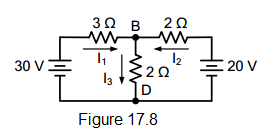electrical circuit figure 17.8

A. 2 A

B. 5 A

C. 2.5 A

D. none of these

View Answer:

Answer: Option B

Solution:

847. The current in 2 Ω horizontal resistor in Fig. 17.8 is _________.electrical circuit figure 17.8

A. 10 A

B. 5 A

C. 2 A

D. 2.5 A

View Answer:

Answer: Option D

Solution:

848. In order to solve the circuit shown in Fig. 17.8 by nodal analysis, we requireelectrical circuit figure 17.8

A. one equation

B. two equation

C. three equations

D. none of these

View Answer:

Answer: Option A

Solution:

849. The superposition theorem is used when the circuit contains

A. a single voltage source

B. a number of passive source

C. passive elements only

D. none of these

View Answer:

Answer: Option B

Solution:

850. Fig. 3.4(b) shows the Thevenin’s equivalent circuit of Fig. 17.9(a). The value of Thevenin’s voltage Vth is ________.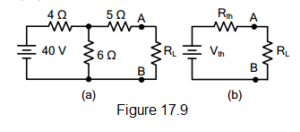electrical circuit figure 17.9

A. 20 V

B. 24 V

C. 12 V

D. 36 V

View Answer:

Answer: Option B

Solution:

#### Questions and Answers in Electrical Circuit

Following is the list of practice exam test questions in this brand new series:

MCQ in Electrical Circuit
PART 1: MCQ from Number 1 – 50                        Answer key: PART 1
PART 2: MCQ from Number 51 – 100                   Answer key: PART 2
PART 3: MCQ from Number 101 – 150                 Answer key: PART 3
PART 4: MCQ from Number 151 – 200                 Answer key: PART 4
PART 5: MCQ from Number 201 – 250                 Answer key: PART 5
PART 6: MCQ from Number 251 – 300                 Answer key: PART 6
PART 7: MCQ from Number 301 – 350                 Answer key: included
PART 8: MCQ from Number 351 – 400                 Answer key: included
PART 9: MCQ from Number 401 – 450                 Answer key: included
PART 10: MCQ from Number 451 – 500                 Answer key: included

### Complete List of MCQ in Electronics Engineering per topic

Please do Subscribe on YouTube!

P inoyBIX educates thousands of reviewers and students a day in preparation for their board examinations. Also provides professionals with materials for their lectures and practice exams. Help me go forward with the same spirit.

“Will you subscribe today via YOUTUBE?”

Subscribe